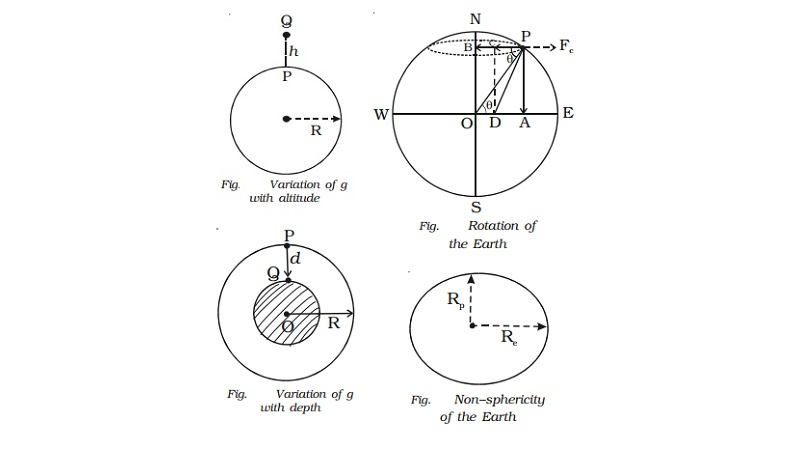Home | | Physics | | Physics | Variation of acceleration due to gravity

# Variation of acceleration due to gravityVariation of acceleration due to gravity : (i) Variation of g with altitude (ii) Variation of g with depth (iii) Variation of g with latitude (iv) Variation of g with latitude (Rotation of the Earth)

Variation of acceleration due to gravity

(i) Variation of g with altitude

Let P be a point on the surface of the Earth and Q be a point at an altitude h. Let the mass of the Earth be M and radius of the Earth be R. Consider the Earth as a spherical shaped body.The acceleration due to gravity at P on the surface is

g =GM/R2  ������.. (1)

Let the body be placed at Q at a height h from the surface of the Earth. The acceleration due to gravity at Q is

gh=GM/(R+h)2    ������.(2)

dividing (2) by (1) gh/g = R2/(R+h)2

By simplifying and expanding using binomial theorem,

gh= g(1-(2h/R))

The value of acceleration due to gravity decreases with increase in height above the surface of the Earth.

(ii) Variation of g with depth

Consider the Earth to be a homogeneous sphere with uniform density of radius R and mass M.Let P be a point on the surface of the Earth and Q be a point at a depth d from the surface.

The acceleration due to gravity at P on the surface is g = g=GM/R2

If ρ be the density, then, the mass of the Earth is M = 4/3    π R3ρ

G= 4/3  G π Rρ

The acceleration due to gravity at Q at a depth d from the surface of the Earth is

gd=GMd2 / (R-d)2

where Md is the mass of the inner sphere of the Earth of radius (R− d).

Md = 4/3   π (R − d)3ρ

gd = 4/3 G π (R − d)ρ

dividing (2) by (1)

gd = R-d/R

gd = g(1-d/R)

The value of acceleration due to gravity decreases with increase of depth.

(iii) Variation of g with latitude (Non−sphericity of the Earth) The Earth is not a perfect sphere. It is an ellipsoid as shown in the Fig.. It is flattened at the poles where the latitude is 90o and bulged at the equator where the latitude is 0o.The radius of the Earth at equatorial plane Re is greater than the radius along the poles Rp by about 21 km.

We know that g = GM/R2

g α 1/R2

The value of g varies inversely as the square of radius of the Earth. The radius at the equator is the greatest. Hence the value of g is minimum at the equator. The radius at poles is the least. Hence, the value of g is maximum at the poles. The value of g increases from the equator to the poles.

(iv) Variation of g with latitude (Rotation of the Earth)

Let us consider the Earth as a homogeneous sphere of mass M and radius R. The Earth rotates about an axis passing through its north and south poles. The Earth rotates from west to east in 24 hours. Its angular velocity is 7.3 � 10−5 rad s−1.Consider a body of mass m on the surface of the Earth at P at a latitude θ. Let ω be the angular velocity. The force (weight) F = mg acts along

PO. It could be resolved into two rectangular components (i) mg cos θ along

PB and (ii) mg sin θ along PA (Fig.).

From the ∆OPB, it is found that BP = R cos θ. The particle describes a circle with B as centre and radius BP = R cos θ.

The body at P experiences a centrifugal force (outward force) FC due to the rotation of the Earth

(i.e) FC = mRω2 cos θ

The net force along PC = mg cos θ − mRω2 cos θ

The body is acted upon by two forces along PA and PC.

The resultant of these two forces is

F= √(mg sinθ) 2+(mg cosθ−mRω2 cosθ)2

The force, F = mg g √[ 2Rω2cos2θ /g]  ����(1)

f g′ is the acceleration of the body at P due to this force F, we have,

F = mg′             ����(2)

by equating (2) and (1)

g′ = g (1-(Rω2cos2θ /g))

Case (i) At the poles, θ = 90o ; cos θ = 0

g′ = g

Case (ii) At the equator, θ = 0 ; cos θ = 1

g′ = g(1- Rω2/g)

So, the value of acceleration due to gravity is maximum at the poles.

Study Material, Lecturing Notes, Assignment, Reference, Wiki description explanation, brief detail

Related Topics